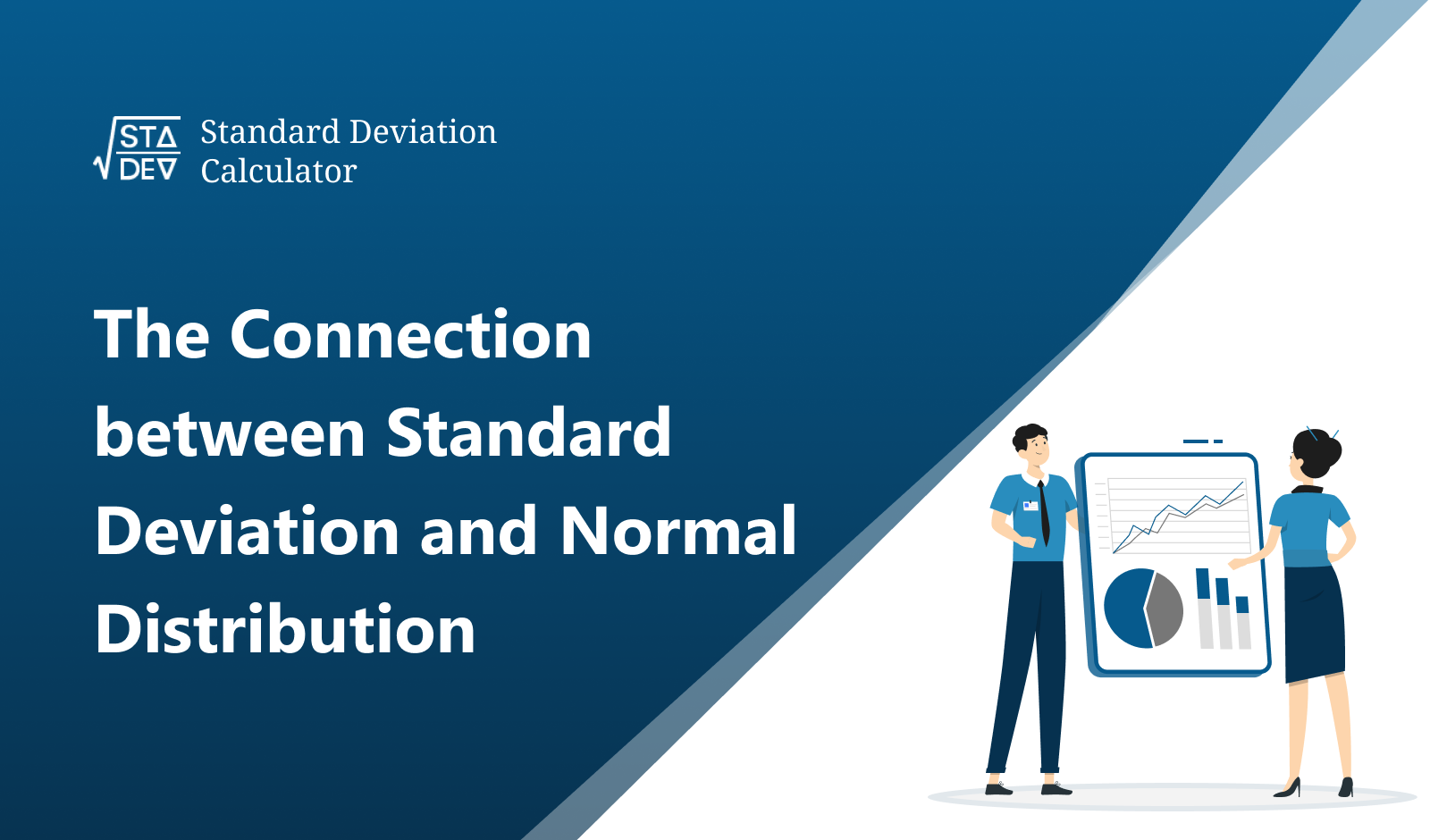Jun 15, 2023
The Connection between Standard Deviation and Normal DistributionNavigating the world of statistics can often seem like traversing an intricate labyrinth. However, when you begin to decipher key elements like Standard Deviation and Normal Distribution, you uncover a fascinating journey through data analysis.

This blog aims to shed light on the intriguing connection between these two statistical concepts and highlight the useful tool Standard Deviation Calculator.

## Shedding Light on Standard Deviation:

In the realm of statistics, Standard Deviation is a cornerstone concept. It's a measurement of how spread-out numbers are from their average or mean.

A lower standard deviation reveals that the data points tend to be closer to the mean. In contrast, a higher standard deviation signifies that data points are spread over a wider range.

Consider a class of students' test scores. If all students score around 85%, the standard deviation is low, indicating minimal variability. But if the scores range from 50% to 100%, the standard deviation is high, demonstrating a higher degree of variability.

## Your Statistical Ally: The Standard Deviation Calculator

Dealing with larger data sets can make a manual computation of standard deviation daunting. This is where the Standard Deviation Calculator becomes your invaluable ally. It instantly computes the standard deviation of any dataset, providing swift and accurate results.

## Decoding Normal Distribution:

Next in our statistical expedition is the concept of Normal Distribution or Gaussian Distribution. It's a bell-shaped, symmetrical pattern of data distribution where the data is centered around the mean.

The normal distribution is shaped by two parameters: the mean (μ), which forms the peak of the curve, and the standard deviation (σ), which defines the width of the bell curve. In a typical normal distribution:

• Approximately 68% of the data falls within one standard deviation from the mean (μ ± σ).
• Roughly 95% falls within two standard deviations (μ ± 2σ).
• Almost 99.7% falls within three standard deviations (μ ± 3σ).

This pattern is often termed the Empirical Rule or the 68-95-99.7 rule.

## The Inseparable Connection: Standard Deviation and Normal Distribution

The interconnection between standard deviation and normal distribution is truly captivating. Essentially, the standard deviation shapes the normal distribution curve. It decides the spread and shape of the curve, essentially defining how much the values within a dataset deviate from the mean.

The following table demonstrates this link vividly:

 Standard Deviation (σ) % of Data Within this Range 1σ (μ ± σ) 68% 2σ (μ ± 2σ) 95% 3σ (μ ± 3σ) 99.7%

## Summing Up:

The enthralling nexus between standard deviation and normal distribution forms the crux of countless statistical analyses. Grasping these concepts and their interconnection is imperative for any data enthusiast. And with tools like the Standard Deviation Calculator, handling these complex computations becomes exponentially easier.

Remember, as you venture through the labyrinth of numbers, the power of standard deviation and normal distribution can be your guiding light, enabling you to sail smoothly through the seas of data.